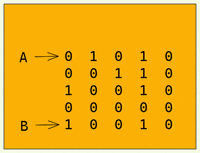# Find the celebrity, Facebook Inteveriew question

Question: Suppose you are at a party with "n" people and among them, there may exist one celebrity. The definition of a celebrity is that all the people at the party know him/her but he/she does not know any of them.

You want to find out who the celebrity is or verify that there is not one. The only thing you are allowed to do is to ask questions like: "Hi, A. Do you know B?" to get information about whether A knows B. You need to find out the celebrity.

Since you don't want to disturb everyone, you want to ask as few questions as possible.

We're given a function

``````knows(a,b) // returns 1 if a knows b or 0.
``````

## Brute Force O(n^2)

Brute Force approach would be to ask everyone, so for a person A we ask if they know everyone else at the party and we do this for each and every guest and on based upon that, we find whos the celebrity.

To implement this, we create two arrays,

``````know[n], doesntKnow[n]
``````

of size n each.

For each person A, we will perform a call,

``````   knows(A,B)
``````

If it returns 1, that means A knows B, so we can do :

``````   know[A] += knows(A,B);             // if A knows B then we add 1 to knows[A]
doesntKnow[B] += knows(A,b);       // if B doesn't know A then we add 0 to doesntKnow[B] or else we add 1
``````

At the, if a guest know[X] = 0 and doestKnow[X] = n-1, we know that X is the celebrity.

Converting it to code:

``````let arr = [[0,0,0,1,0],
[0,0,0,1,0],
[0,0,0,1,0],
[0,0,0,0,0],
[0,0,0,1,0]];

function knows(i,j){
return arr[i][j];
}

function celebrity(n){
let know = new Array(n).fill(0);
let doesntKnow = new Array(n).fill(0);

for(let i=0;i<n;i++){
for(let j=0;j<n;j++){
know[j] += knows(i,j);
doesntKnow[i] += knows(i,j);
}
}

for(let i=0;i<n;i++){
if(know[i] == n-1 && doesntKnow[i] == 0){
return i;
}
}
return -1;
}
``````

Now, let's think about optimizing this.

All we care about is whether person A knows person B. If person A knows B then A can't be the celebrity or if B knows person A then B can't be the celebrity since B doesn't know anyone.

Based on this simple observation, let's optimize our solution.
First : We shall eliminate those who know someone.
Second : When we get to a point where there's only one person remaining we check if that person knows anyone else ? If not then that person is the celebrity.

So how shall we eliminate those who know someone else?
We shall maintain two pointers, let A : 0 and B : N. then we ask know(A,B),
if it returns true then we move pointer A : 1, or else we move pointer B : N-1.

To understand it clearly see this gif :After this we reach will reach check if the Pointer A, is really indeed a celebrity by checking
1> Celebrity doesn't know anyone else :

``````   for i = 0 -> N;
knows(A,i)  // should be false
``````

2> Everyone else knows the celebrity :

``````   for i = 0 -> N;
knows(i,A) // should be true
``````

Putting it all together :

``````  let arr = [[0,1,0,1,0],
[1,0,0,1,0],
[0,0,0,0,0],
[0,0,0,0,0],
[0,1,0,1,0]];

function knows(i,j){
return arr[i][j];
}

function celebrity(n){

let A = 0;
let B = n-1;

while(A<B){
if(knows(A,B)){
A++;
}else{
B--;
}
}

for(let i=0;i<n;i++){
if( i!=A && (knows(A,i) || !knows(i,A))){
return -1;
}
}

return A;
}
``````

I hope you liked my explanation :)